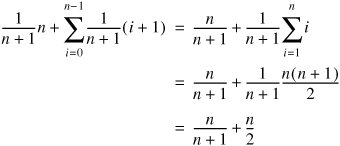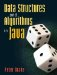# Linear Search

Suppose we want to determine whether an array of ints contains some target number. The obvious approach is the one used in the contains() method of the ArrayList class (Section 5.3): start at the beginning and examine each element in turn. This algorithm is called linear search. Not surprisingly, it takes linear time in both the worst and average cases.

We can make the algorithm slightly more efficient if we know in advance that the array is sorted. The numbers we encounter during a search increase as we move from left to right across the array. If we ever encounter a number which is larger than the target, we can stop searching. Since all of the remaining numbers must be even larger, the target can't possibly appear later in the array.

The code for this improved linear search is given in Figure 8-1.

Figure 8-1. If the array data is already sorted, a linear search can sometimes be stopped early.

 ```1 /** Return true if target appears in the sorted array data. */ 2 public static boolean linearSearch(int[] data, int target) { 3 for (int i = 0; (i < data.length) && (data[i] <= target); i++) { 4 if (data[i] == target) { 5 return true; 6 } 7 } 8 return false; 9 } ```

In the worst case, this is no faster than the old version. On average, although the running time is still in Q(n), the number of elements we have to examine in a successful search is reduced by a factor of 2. A formal proof of this is left as Exercise 8.2.

To analyze the average number of passes through the loop on an unsuccessful search, we define an exhaustive set of mutually exclusive events. Let event i be the event that the target, if it were present, would belong right before element i. There is one event for each of the n numbers (events 0 through n 1), plus event n, where the target is larger than anything in the array.

In event n, we have to examine n elements to determine that the target is not present. In events 0 through n 1, we have to examine i + 1 elements. If we assume that the n + 1 events are equally likely, the average number of elements examined is:Thus, on average, we only have to look at between n/2 and (n/2) + 1 elements.

Exercises

 8.1 There are n! permutations of a set of n elements. For example, the set {A, B, C} has 3! = 6 permutations: ABC, ACB, BAC, BCA, CAB, and CBA. There are (n + 1)! permutations of the set after we add a new target. Argue that, if each of these permutations is equally likely, each of the n + 1 places where the target might belong is equally likely. 8.2 Analyze the average time for a successful linear search.

### Binary SearchData Structures and Algorithms in Java
ISBN: 0131469142
EAN: 2147483647
Year: 2004
Pages: 216
Authors: Peter Drake